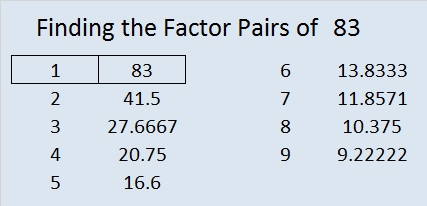# 83 and Level 1This week’s puzzles and last week’s solutions: 12 Factors 2014-04-07

• 83 is a prime number.
• Prime factorization: 83 is prime.
• The exponent of prime number 83 is 1. Adding 1 to that exponent we get (1 + 1) = 2. Therefore 83 has exactly 2 factors.
• Factors of 83: 1, 83
• Factor pairs: 83 = 1 x 83
• 83 has no square factors that allow its square root to be simplified. √83 ≈ 9.11043How do we know that 83 is a prime number? If 83 were not a prime number, then it would be divisible by at least one prime number less than or equal to √83 ≈ 9.1. Since 83 cannot be divided evenly by 2, 3, 5, or 7, we know that 83 is a prime number.

83 is never a clue in the FIND THE FACTORS puzzles.

This site uses Akismet to reduce spam. Learn how your comment data is processed.